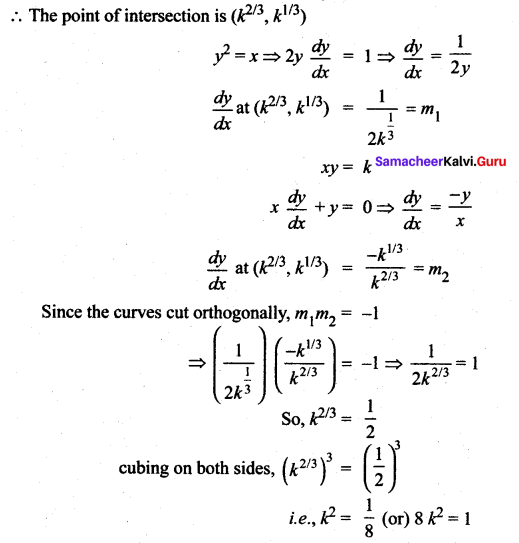Samacheer Kalvi 12th Maths Solutions Chapter 7 Applications of Differential Calculus Ex 7.2

Tamilnadu Samacheer Kalvi 12th Maths Solutions Chapter 7 Applications of Differential Calculus Ex 7.2

Question 1.
Find the slope of the tangent to the curves at the respective given points.
(i) y = x4 + 2x2 – x at x = 1
(ii) x = a cos3 t, sin 3 t at t = $$\frac{\pi}{2}$$
Solution:
(i) y = x4 + 2x2 – x at x = 1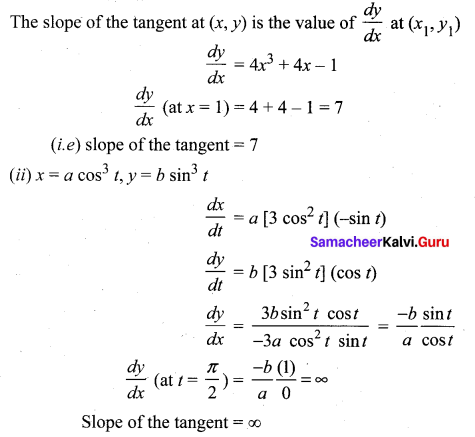Question 2.
Find the point on the curve y = x2 – 5x + 4 at which the tangent is parallel to the line 3x + y = 7.
Solution:
Let (x1, y1) be the required point.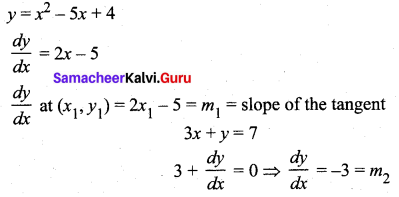Given tangent is similarly to the line ⇒ m1 = m2
⇒ 2x1 – 5 = -3
⇒ 2x1 = -3 + 5 = 2
⇒ x1 = 1
Substituting x1 = 1 in the curve.
y1 = 1 – 5 + 4 = 0
So the required point is (1, 0)Question 3.
Find the points on the curves = x3 – 6x2 + x + 3 where the normal is parallel to the line x + y = 1729.
Solution:
Let (x1,y1) be the required point
y = x3 – 6x2 + x + 3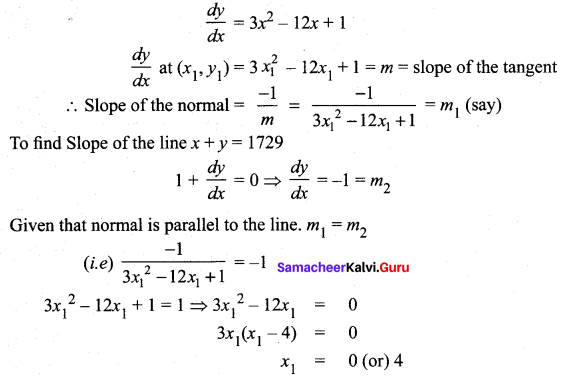Substituting x1 values in the curve
when x1 = 4, y1 = 3
when x1 = 4, y1 = 43 – 6(4)2 + 4 + 3 = 64 – 96 + 4 + 3 = -25
So the required points are (0, 3) and (4, -25)

Question 4.
Find the points on the curve y2 – 4xy = x2 + 5 for which the tangent is horizontal.
Solution: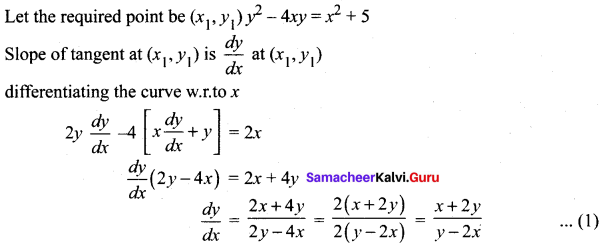Given that tangent is horizontal (i.e) tangent is parallel to x – axis
⇒ Equation of tangent will be of the form y = c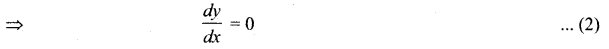from (1) and (2) we get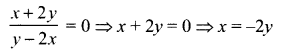Substituting x = -2y in the equation of the curve we get
y2 – 4(-2y)(y) = 4y2 + 5
⇒ y2 + 8y2 – 4y2 = 5
⇒ 5y2 = 5 ⇒ y2 = 1
⇒ y = ± 1
when y = 1, x = -2 and when y = -1, x = 2 So the points are (2, -1), (-2, 1)

Question 5.
Find the tangent and normal to the following curves at the given points on the curve.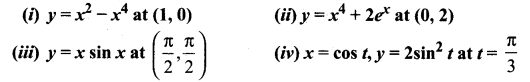Solution: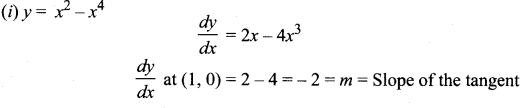Now m = -2, (x1, y1) = (1, 0)
So equation of the tangent is
y – y1 = m(x – x1)
(i. e) y – 0 = -2(x – 1)
y = -2x + 2
2x+ y = 2
Slope of tangent = m = -2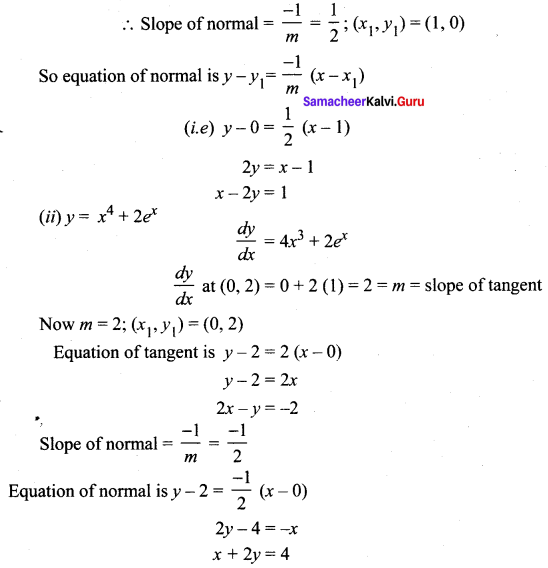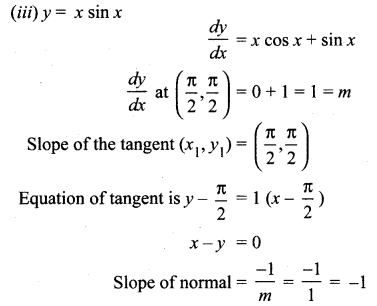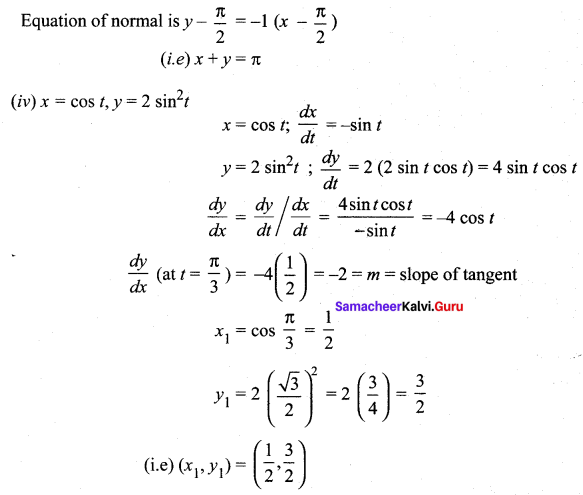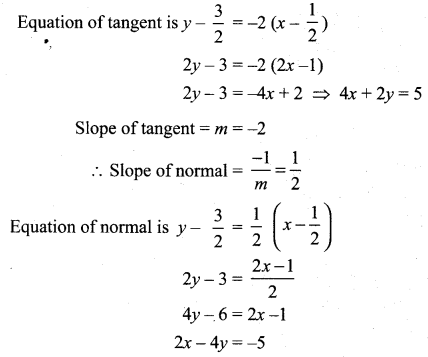Question 6.
Find the equations of the tangents to the curve y = 1 + x3 for which the tangent is orthogonal with the line x + 12y = 12.
Solution: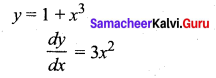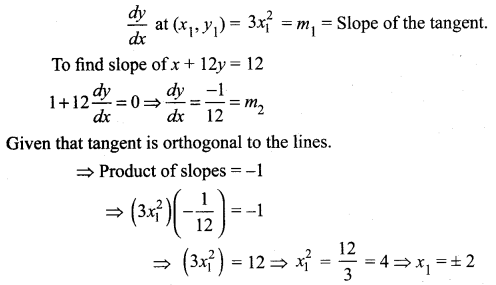Substituting x1 values in the curve
when x1 = 2, y1 = 9; when x1 = -2, y1 = -1
So the points are (2, 9) and (-2, -7)
To find the equations of tangents:
Tangents are orthogonal to x + 12y = 12
So equations of tangents will be of the form 12x – y = k
The tangent passes through (2, 9) ⇒ 24 – 9 = k ⇒ k = 15 .
∴ Equation of tangent is 12x – y = 15
The tangent passes through (-2, -7) ⇒ 12 (-2) + 7 = k ⇒ -17
So equation of tangent is 12x – y = -17

Question 7.
Find the equations of the tangents to the curve y = $$\frac{x+1}{x-1}$$which are parallel to the line x + 2y = 6.
Solution: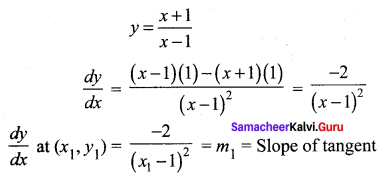Tangent is parallel the line x + 2y = 6
⇒ Slope of tangent = Slope of line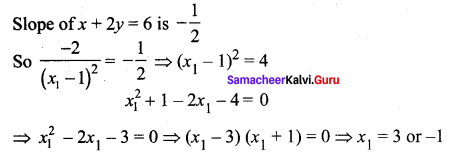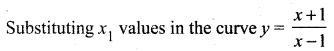when x1 = -1, y1 = 0; when x1 = 3, y1 = 2
So the points are (-1, 0) and (3, 2). The tangents are parallel to x + 2y = 6. So equation of tangents will be of the form x + 2y = k.
∴ Equation of tangent is x + 2y = -1
The tangent passes through (-1, 0) ⇒ -1 = k
The tangent passes through (3, 2) ⇒ 3 + 4 = k ⇒ k = 7
∴ Equation of tangent is x + 2y = 7

Question 8.
Find the equation of tangent and normal to the curve given by x = 7 cos t and y = 2sin t, t ∈ R at any point on the curve.
Solution: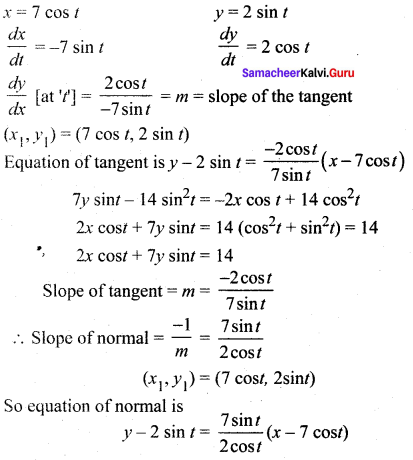(2 cos t)y – 4 sint cos t = (7 sin t)x – 49 sin t cos t
x(7 sin t) – y(2 cos t) = 45 sin t cos t

Question 9.
Find the angle between the rectangular hyperbola xy = 2 and the parabola x2 + 4y = 0.
Solution:
Solving the given two equations. To find the point of intersection: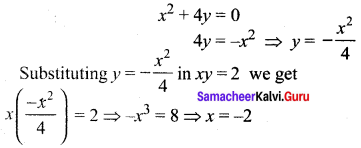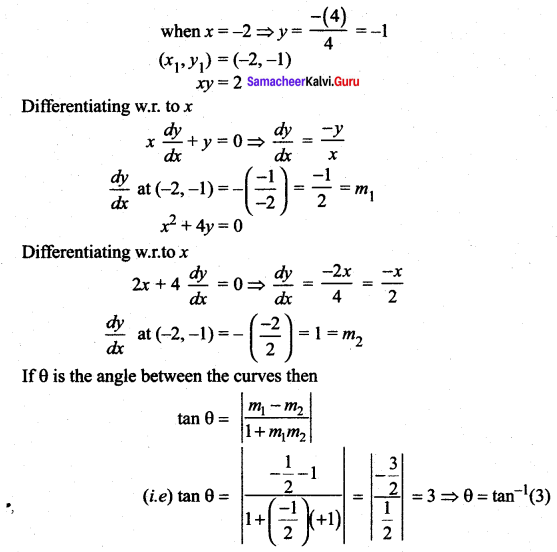Question 10.
Show that the two curves x2 – y2 = r2 and xy = c2 where c, r are constants, cut orthogonally.
Solution:
Let (x1, y1) be the point of intersection of the two curves
I Curve: x2 – y2 = r2
Differentiating w.r.to x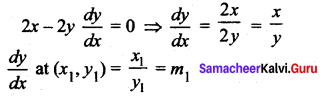II Curve: xy = c2
Differentiating w.r.to x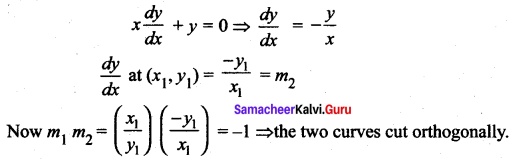Samacheer Kalvi 12th Maths Solutions Chapter 7 Applications of Differential Calculus Ex 7.2 Additional Questions

Question 1.
Prove that the sum of the intercepts on the co-ordinate axes of any tangent to the curve x = a cos4 θ, y = a sin4 θ, 0 < θ < $$\frac{\pi}{2}$$ is equal to a.
Solution: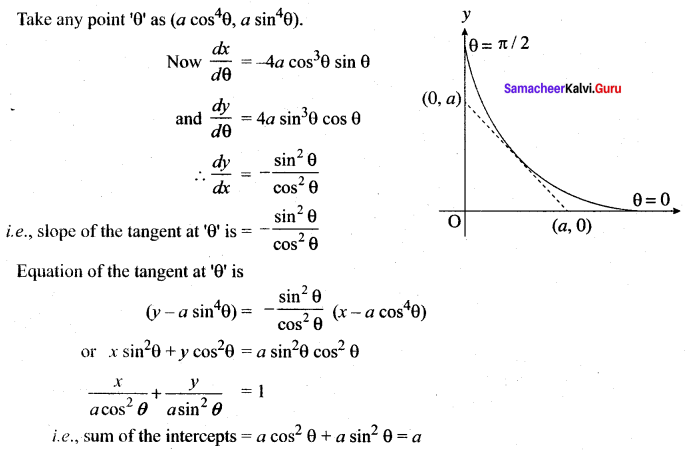Question 2.
Find the equations of a normal to y = x3 – 3x that is parallel to 2x + 18y – 9 = 0.
Solution:
Let (x1, y,) be a point on the curve.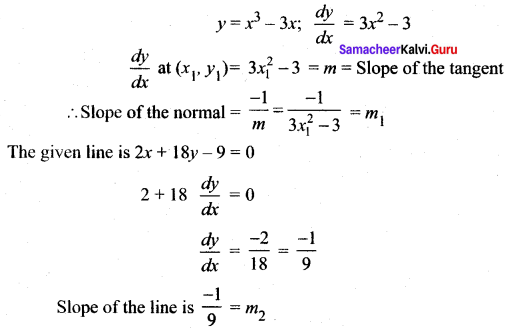It is given that the normal is parallel to the line ⇒ m1 = m2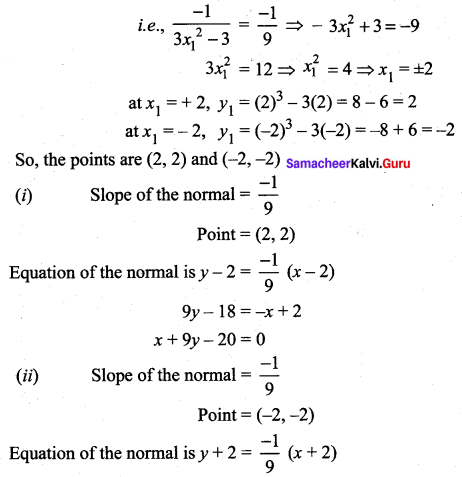9y + 18 = -x – 2
x + 9y + 20 = 0

Question 3.
Prove that the curves 2x2 + 4y2 = 1 and 6x2 – 12y2 = 1 cut each other at right angles.
Solution:
Solving the given two equations,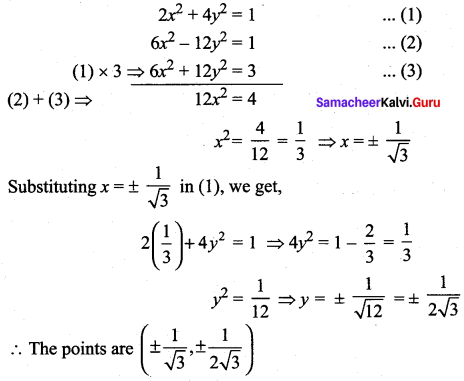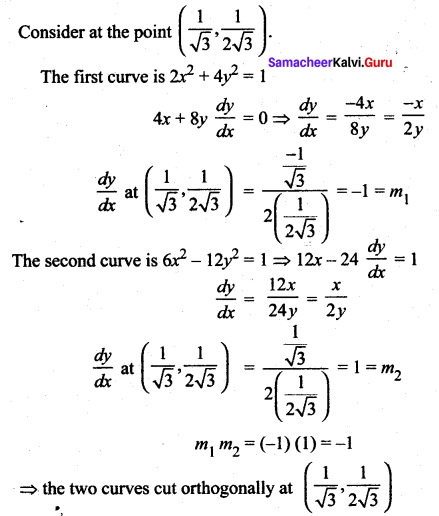Similarly it can be proved at the other points also.

Question 4.
Show that the equation of the normal to the curve x = a cos3 θ, y = a sin3 θ at ‘θ’ is x cos θ – y sin θ = a cos 2θ
Solution: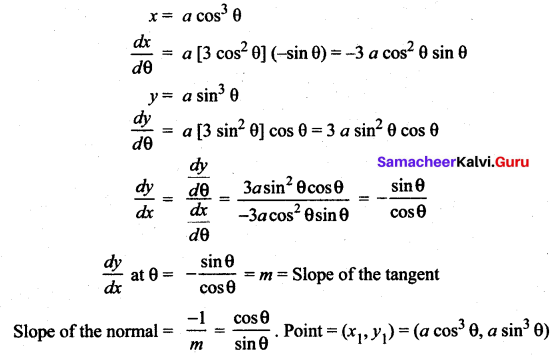So, equation of the normal is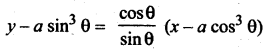i.e. y sin θ – a sin4 θ = x cos θ – a cos4 θ
x cos θ – y sin θ = a cos4 θ – a sin4 θ
i.e.x cos θ – y sin θ = a[cos2 θ + sin2θ][cos2θ – sin2 θ]
= a[cos 2 θ]
So, the equation of the normai is x cos θ – y sin θ = a cos 2θQuestion 5.
If the curve y2 = x and xy = k are orthogonal, then prove that 8k2 = 1.
Solution:
y2 = x, xy = k
Solving the two equations, we get, (y2) (y) = k
y3 = k